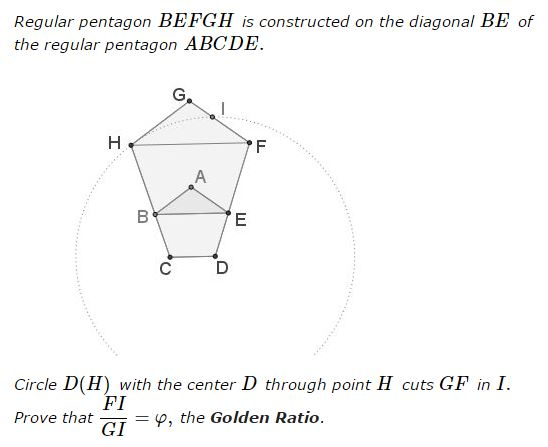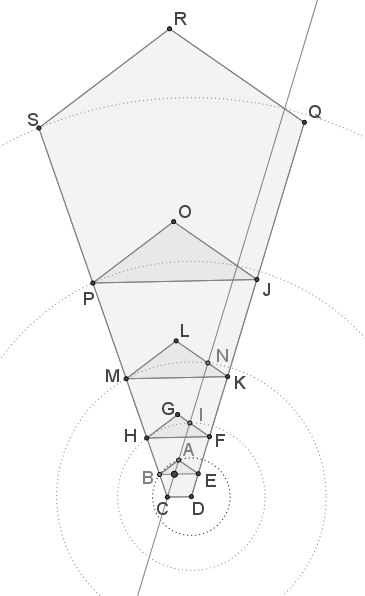# Cultivating Regular Pentagons

### Problem### Solution 1

First note that, being an isosceles trapezoid, $CDFH\,$ is cyclic. Let $I'\,$ be the point where circle $(CDFH)\,$ meets $FG.$In the regular pentagon $ABCDE,\,$ $AC\parallel DE,\,$ and let the extension of $AC\,$ cross $FG\,$ in $I''.\,$ We'll show that $I'=I''=I.$

Trapezoid $CDFI''\,$ is isosceles, hence, cyclic. $(CDFI'')=(CDF)=(CDFH)\,$ from which $I''=I'.\,$ Further, in the isosceles trapezoid $CDFI''\,$ $CF=DI'';\,$ in the isosceles trapezoid $CDFH\,$ $CF=DH.\,$ By transitivity, $DI''=CF=DH,\,$ such that $I''\in D(H)\,$ and, subsequently, $I''=I.$

To solve the original problem, suffice it to note that $AC\,$ divides $BE\,$ in the golden ratio. Thus, by Thales' theorem, it is also true for $FG:$### Solution 2

Let $\beta=72^o\,$ and the side of the smaller pentagon be $p.\,$ Let $\displaystyle k=\frac{\varphi}{1+\varphi}.$

The side of the larger pentagon is then $q=2p\cos(\beta/2).\,$ With origin at $D\,$ and the $X\,$ axis parallel to $CD,\,$ the coordinates of the relevant points are:

\displaystyle \begin{align} F&\rightarrow \left((p+q)\cos\beta,(p+q)\sin\beta\right), \\ H&\rightarrow \left(-p-(p+q)\cos\beta,(p+q)\sin\beta\right), \\ G&\rightarrow \left((p+q)\cos\beta+q\cos 2\beta,(p+q)\sin\beta+q\sin 2\beta\right), \\ I&\rightarrow \left((p+q)\cos\beta+kq\cos 2\beta,(p+q)\sin\beta+kq\sin 2\beta\right). \end{align}

Both, $H\,$ and $I\,$ being on the circle centered at $D\,$ means

\displaystyle \begin{align} &|I|^2=|H|^2 \\ &[(p+q)\cos\beta+kq\cos 2\beta]^2+[(p+q)\sin\beta+kq\sin 2\beta]^2 = \\ &\qquad\qquad\qquad[-p-(p+q)\cos\beta]^2+[(p+q)\sin\beta]^2 \\ &k^2q^2+2kq(p+q)\cos\beta-p^2-2p(p+q)\cos\beta=0 \\ &4k^2\cos^2\left(\frac{\beta}{2}\right)+4\cos\left(\frac{\beta}{2}\right)k\left[1+2\cos\left(\frac{\beta}{2}\right)\right]\cos\beta-1 \\ &\qquad\qquad\qquad-2\left[1+2\cos\left(\frac{\beta}{2}\right)\right]\cos\beta=0 \end{align}

Plugging in the values:

\displaystyle \begin{align} \cos\left(\frac{\beta}{2}\right)&= \cos~36^o=\frac{\sqrt{5}+1}{4} \\ \cos\beta&= \cos~72^o=\frac{\sqrt{5}-1}{4}, \end{align}

gives

$\displaystyle 2(\sqrt{5}+3)k^2+(\sqrt{5}+1)^2k-2(\sqrt{5}+3)=0$

with roots

$\displaystyle k=\left\{\left(\frac{\sqrt{5}-1}{2}\right),-\left(\frac{\sqrt{5}+1}{2}\right)\right\}.$

Choosing the physical root,

\displaystyle \begin{align} \frac{\varphi}{1+\varphi}&= \frac{\sqrt{5}-1}{2}, \\ \varphi&= \frac{\sqrt{5}+1}{2}. \end{align}

### Extension

We may continue building a pyramid of regular pentagons, as shown below:As above, the circles $D(M),\,\,$ $D(P),\,\,$ $D(S),\,\,$ etc., meet $AC\,\,\,$ on the sides of the corresponding pentagons. If $N=AC\cap KL=D(M)\cap KL,\,\,$ then $\displaystyle \frac{LN}{KN}=\varphi,\,\,$ the Golden Ratio.

### Acknowledgment

The problem has been kindly posted by Tran Quang Hung at the CutTheKnotMath facebook page. GeoGebra made it easy to draw and investigate the construction. Solution 2 is by Amit Itagi.

### Golden Ratio